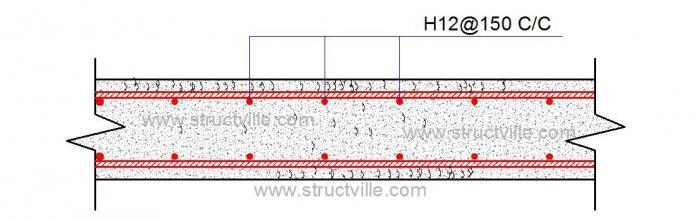# Minimum Area of Steel for Imposed Deformation CrackingMinimum reinforcement is provided to ensure that yielding does not occur, and by so doing, cracking is adequately controlled in a concrete section. The aim of the calculation is to obtain the minimum area of steel that is required to prevent early thermal cracking in a concrete section, especially for water retaining structures. This minimum area of reinforcement for imposed deformation cracking is different from the minimum area of reinforcement required for actions according to detailing guidelines. The difference lies in the stress distribution factor kc.

Minimum area of reinforcement required for imposed deformation cracking is given in equation (7.1) of in BS EN 1992-1-1.

As,min = kck Act ( fct,eff /fyk)

Where;
kc = A coefficient to account for stress distribution
= 1.0 for pure tension
= 0.4 for pure bending.

When cracking first occurs the cause is usually early thermal effects and the whole section is likely to be in tension, so take kc = 1.0.

k = A coefficient to account for self-equilibrating stresses
= 1.0 for thickness h < 300 mm and 0.65 for h > 800 mm

(interpolation is allowed for thicknesses between 300 mm and 800 mm).

Act = area of concrete in the tension zone just prior to onset of cracking.
Act is determined from section properties but generally for basement slabs and walls is most often based on full thickness of the section.

fct,eff  = fctm = mean tensile strength when cracking may be first expected to occur:

for early thermal effects 3 days
for long-term effects, 28 days (which is considered to be a reasonable approximation)

For example, to calculate the tensile strength of C30/37 concrete at t = 3 days using class R cement.

s = 0.20 for Class R cement
t = 3 days;

βcc(3) = exp{s [1 − √ (28/t)]} = 0.663

fctm for C30 = 2.89 MPa (using the relation fctm = 0.3fck2/3)
fct,eff = fctm = βcc(3)(fctm) = 0.663 × 2.89 = 1.916 Mpa

See Table 1 below for typical values of mean tensile strength at different ages of concrete.

Table 1: Typical values of mean tensile strength of concrete

#### Solved Example

Calculate the minimum area of steel required for a concrete slab 400 mm thick against early thermal cracking. The concrete slab is to be done using class N cement (fyk = 500 MPa).

To obtain the value of k, we have to interpolate between for h = 400 mm (between 300 mm and 800 mm)

k = 1 + {(400 – 300)/(800 – 300)] × (0.65 – 1.0) = 0.93

Act = area of concrete within tensile zone
Also note that tensile zone is that part of the section which is calculated to be in tension just before formation of the first crack.

For this example;
kc = 1.0
k = 0.93 (from interpolation)

At 3 days early cracking, fct,eff  = 1.73 MPa (class N cement)
σs = Stress in the reinforcement (fyk) = 500 Mpa
Act = 400 × 1000 = 4 × 105 mm2

Therefore;

As,min = (0.93 × 1.0 × 1.73 × 4 × 105)/500 = 1287 mm2

Provide at each face H12@175 c/c (Asprov = 646 mm2/m)

Total area of steel provided in the section = 2 × 646 = 1292 mm2/m > 1287 mm2    Ok

Note that the provision of minimum reinforcement does not does not guarantee design crack width. Additional calculations will need to be done to calculate the crack width due to early thermal cracking, long term cracking, and flexural cracking.